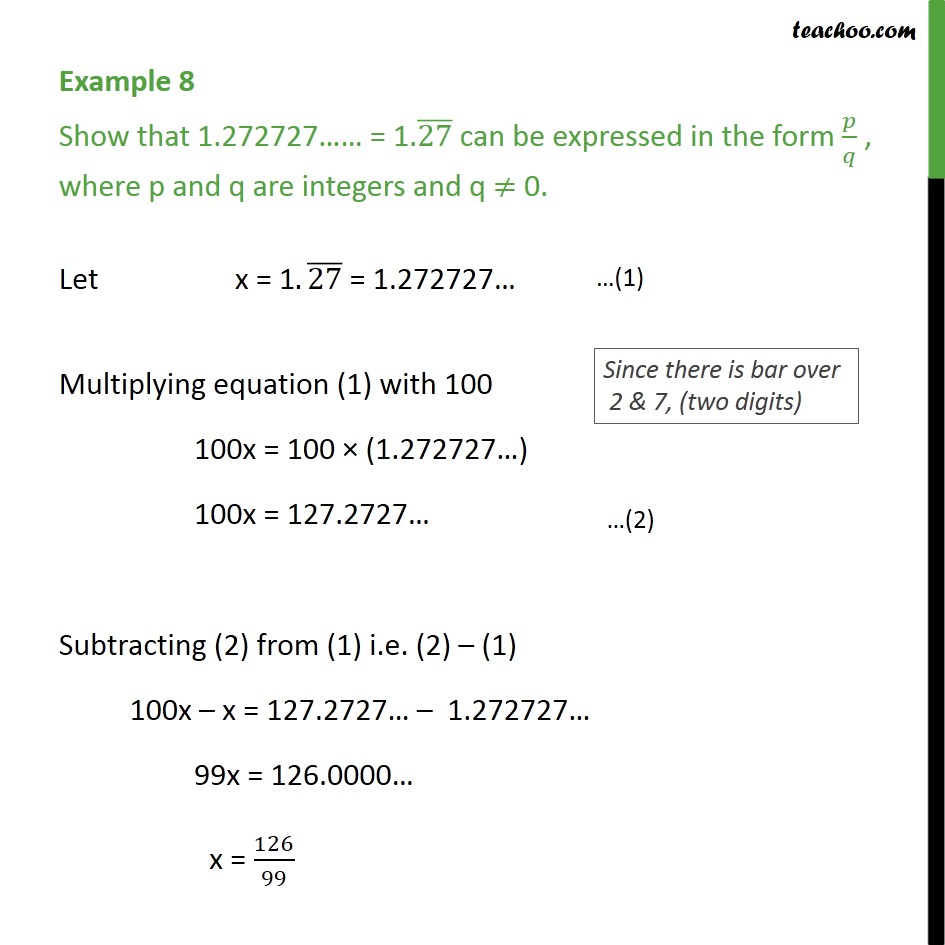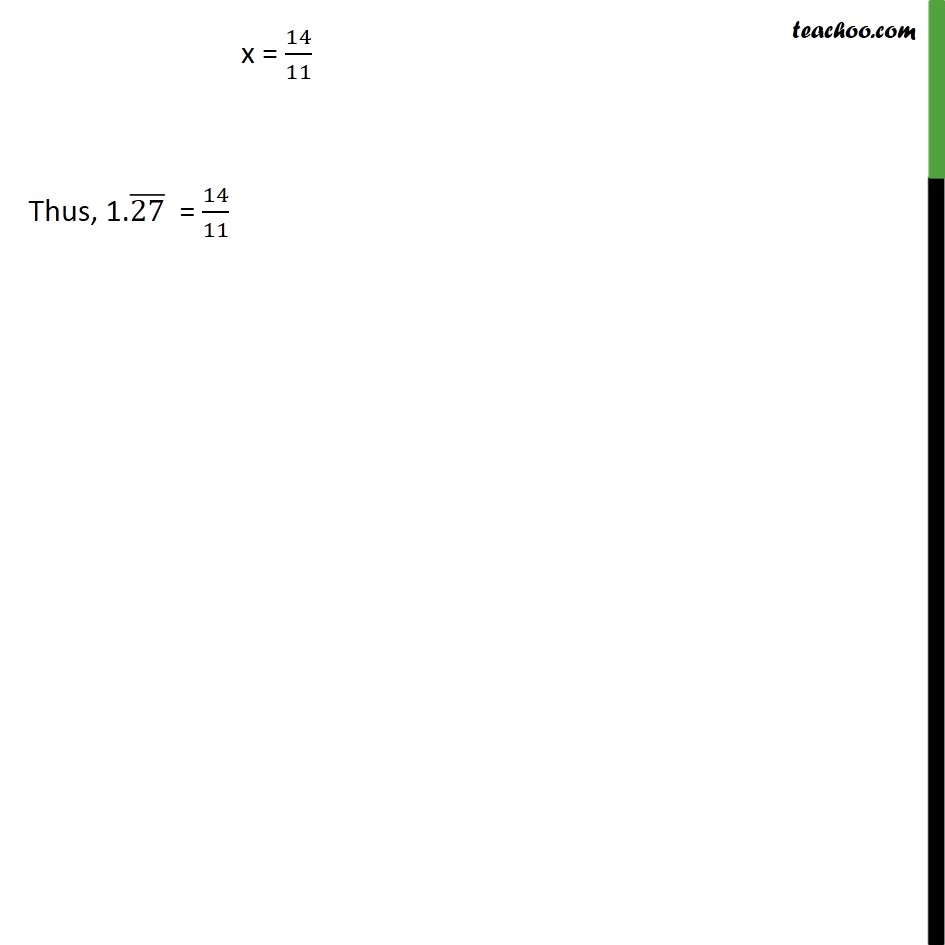1. Chapter 1 Class 9 Number Systems
2. Concept wise
3. Expressing decimal in p/q

Transcript

Example 8 Show that 1.272727 = 1.(27) can be expressed in the form / , where p and q are integers and q 0. Let x = 1.(27) = 1.272727 Multiplying equation (1) with 100 100x = 100 (1.272727 ) 100x = 127.2727 Subtracting (2) from (1) i.e. (2) (1) 100x x = 127.2727 1.272727 99x = 126.0000 x = 126/99 x = 14/11 Thus, 1.(27) = 14/11

Expressing decimal in p/q